# Notes on Opamp Bandwidth

Opamp datasheets usually provide certain parameters to specify the frequency response of the opamp. An often misunderstood spec is on the Gain Bandwidth Product. I have traditionally had a poor understanding of this subject matter; hope this post helps clear things up for those in similar shoes.

The transfer function of a typical opamp has a real dominant pole at a fairly low frequency (a few Hertz). The opamp’s transfer function can be approximated by:

$$A_0$$ is the DC gain and $$\omega_0$$ is the dominant pole frequency (which is also referred as the $$-3dB$$ bandwidth of the opamp). The gain of the opamp at frequency $$\omega$$ can then be calculated as:

Gain Bandwidth Product is usually defined for dominant pole compensated opamps. For frequencies beyond $$-3dB$$ frequency ($$\omega_0$$), the product of gain and frequency is constant. Unity Gain Bandwidth and Gain Bandwidth Product have the same value for a dominant pole compensated opamp. ie, $$GBWP = A_0 \omega_0$$. Shown below is the Bode plot of the frequency response of the opamp discussed above.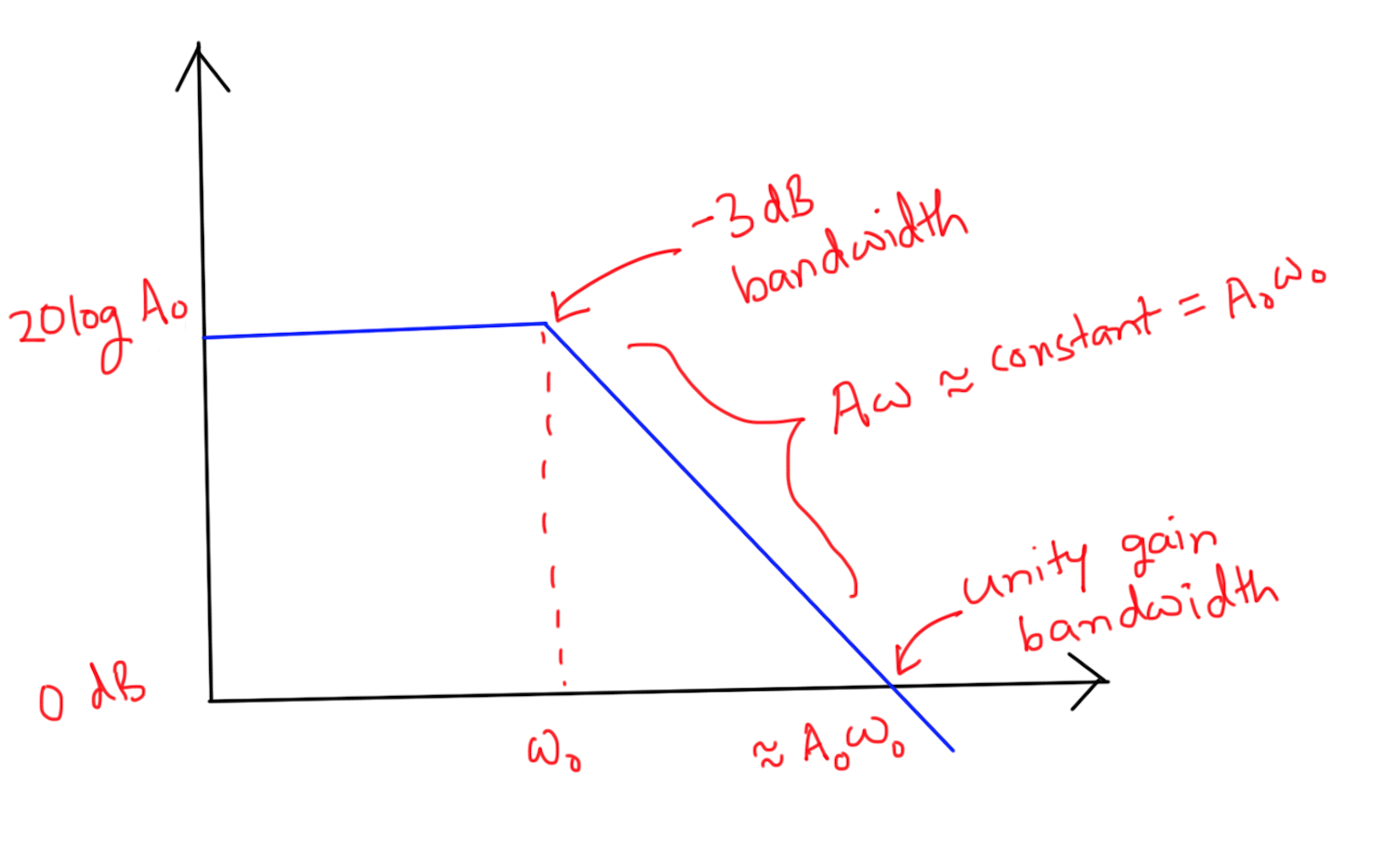If we were to plot the Gain vs Frequency graph on a linear scale (instead of the log-log scale as in the Bode Plot), the curve would approximate a hyperbola (a bit beyond $$\omega_0$$).

## GBWP of non-inverting amplifier

There is yet another way to interpret the GBWP of opamps. Here is the classic non-inverting amplifier configuration.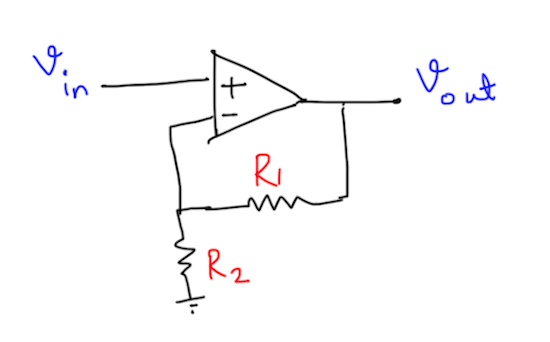We usually come across statements which say that the $$-3dB$$ bandwidth of this amplifier is GBWP divided by Gain. However, this statement is not quite obvious. Let us formally prove that this is indeed the case. At a block diagram level, this circuit can be equivalently represented as follows: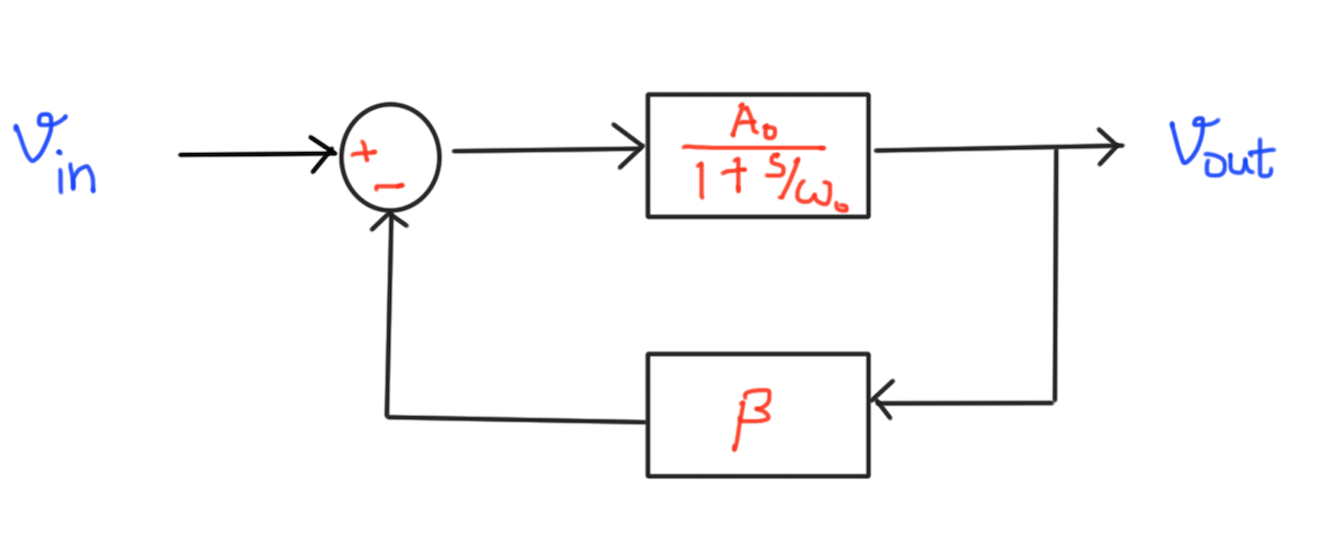where $$\beta = \frac{R_2}{R_1 + R_2}$$. The closed loop transfer function of this new system is

Observe that $$A_0 \omega_0 = A_1 \omega_1$$. The graph below shows the effect of the $$\beta$$ on the Gain Bode Plot of the amplifier.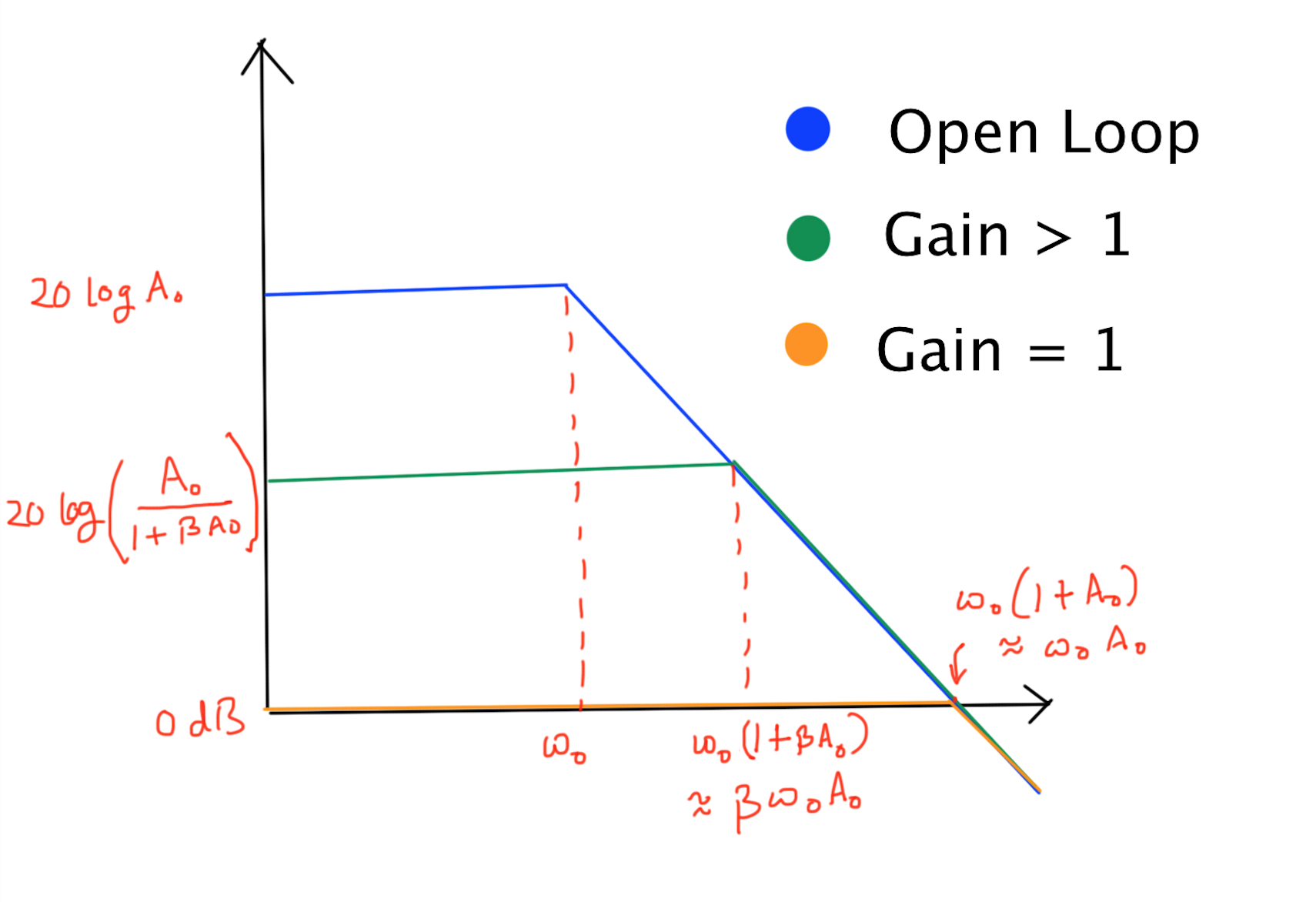## Bandwidth of Cascaded Opamps

Consider $$n$$ identical cascaded opamps, where each of the opamp has a resistive feedback loop with a certain $$\beta$$. If the GBWP of the opamp is known, then can we somehow predict the GBWP of the cascaded system?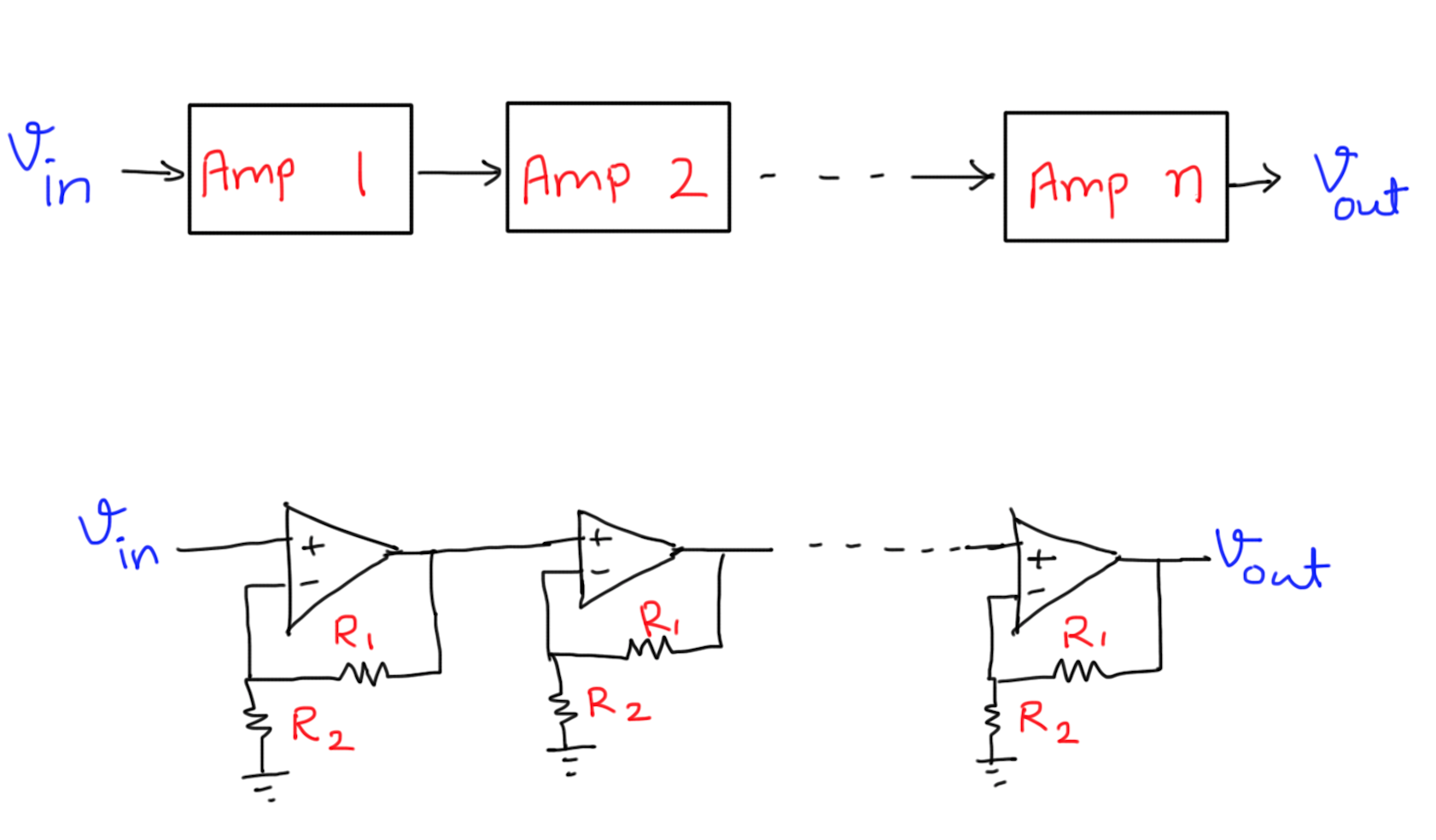Each of the amplifier has a GBWP as $$A_0 \omega_0$$, and since the gain of each amplifier stage is determined by $$R_1$$ and $$R_2$$, (gain = $$K = A_0 \frac{R_1 + R_2}{R2}$$) => the bandwidth of each amplifier stage is $$\omega_0 \frac{R_2}{R_1 + R_2}$$. On first look, it appears that the bandwidth of the cascaded system should also be $$\omega_0 \frac{R_2}{R_1 + R_2}$$, but that is not exactly the case. The straight line approximation in bode plot is not good enough in this case! Let us find out the true $$-3dB$$ bandwidth.

Say we have a specific opamp with a $$1 MHz$$ GBWP. If we were to use this opamp to get a gain of $$1000$$, the $$-3dB$$ bandwidth would drop to around $$1 kHz$$. Instead, if we use $$3$$ cascaded opamps (each with a gain of $$10$$, and hence, an individual bandwidth of $$100 kHz$$), the cumulative bandwidth of the cascaded system would be

That’s it for today!

comments powered by Disqus